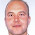# Learning Clojure

## Thursday, July 11, 2013

### Randomized Quicksort

```;; Randomized Quicksort

;; Quicksort has very unfortunate performance characteristics. It's
;; very fast on randomized data, and uses very little extra memory,
;; but if there's too much order in its input, it becomes a quadratic
;; algorithm which blows the stack.

;; Such inputs are very rare, but very easy to find (there's glory for you!)

;; Luckily there's an easy fix, which is why Quicksort's a very popular sorting algorithm.

;; Here's Quicksort as we left it:

(defn swap [v i1 i2]
(assoc v i2 (v i1) i1 (v i2)))

(defn ^:dynamic partition-by-pivot [v start end]
(loop [v v i (inc start) j (inc start)] ;; this extra loop just sets up the indices i and j
(if (> j end)
[(dec i) (swap v start (dec i))]
(if (< (v start) (v j))
(recur v i (inc j))
(recur (swap v i j) (inc i) (inc j))))))

(defn ^:dynamic qsort
([v start end]
(if (> (+ 1 start) end) v  ;; short array, nothing to do
(let [[index newv] (partition-by-pivot v start end) ;; otherwise partition
leftsorted (qsort newv start (dec index))] ;; and sort the left half
(qsort leftsorted (inc index) end)))))

(defn quicksort [v] (let [vec (into [] v)] (qsort vec 0 (dec (count vec)))))

;; Potentially a very fast sorting algorithm, which apart from its recursion stack
;; needs no extra memory.

;; But although it reliably looks good on random input:
(time (first (quicksort (shuffle (range 2048))))) "Elapsed time: 435.670347 msecs"

;; If you feed it an array which is already in order:
(time (first (quicksort (range 2048)))) "Elapsed time: 2413.521649 msecs"
;; performance drops off horribly
(time (first (quicksort (range 4096))))
;; and the stack blows.

;; The underlying problem is that when the data has a structure which makes the pivots
;; far from the medians of the arrays to be partitioned, the recursion tree unbalances, and
;; rather than doing roughly log 2048=11 recursions, quicksort does 2048 recursions.

;; If only we could get the usual performance characteristics of quicksort on random data
;; on the sort of almost-sorted data that we often encounter in practice.

;; One way to do this, of course, would be to shuffle the data before sorting it.
;; The chances of hitting a bad case with properly shuffled data are astronomically small.
;; It would work, but isn't that an odd thing to do? And shuffling is just as difficult as sorting.

;; Turns out we don't have to. If we pick the pivot at random, then we get the same performance.
;; There are still bad cases, but if the random number generator is uncorrelated with the data to
;; be sorted, we'll never hit one in a million years.

;; How shall we do that? Turns out it's dead easy to frig
;; partition-by-pivot. We just swap the first element of the array
;; with a randomly chosen one before we pick the pivot:

(defn ^:dynamic partition-by-pivot [v start end]
(let [randomel (+ start (rand-int (- end start)))
v (swap v start randomel)] ;; swap first element with randomly chosen one
(loop [v v i (inc start) j (inc start)]
(if (> j end)
[(dec i) (swap v start (dec i))]
(if (< (v start) (v j))
(recur v i (inc j))
(recur (swap v i j) (inc i) (inc j)))))))

(time (first (quicksort (range 2048)))) "Elapsed time: 666.069407 msecs"

;; The bad cases are still around. But they're very hard to find now.

;; It occurs to me that one way to blow up randomized quicksort might
;; be to use a pseudo-random number generator and a well chosen
;; algorithm to shuffle the data, and then use the same PRNG to
;; quicksort it. So if you've got a clever adversary who knows what
;; you mean by random he should be able to make your quicksorts
;; explode.

;; We're something like a factor of twenty away from clojure's built in sort.
;; That's really not bad for an unoptimized algorithm.
(time (first (sort (shuffle (range 2048))))) "Elapsed time: 33.509778 msecs"

;; It should be possible to speed our quicksort up quite a bit (especially if
;; we restrict ourselves to arrays of integers).

;; I leave this as an exercise for the reader.

```

1.Hi,

Just wanted to say thanks for the great Clojure blog and share with you my qsort. Sorry for the bad indentation...

(defn qsort [s]
(lazy-seq
(when-let [[x & xs] s]
(let [{:keys [lt gt]} (group-by #(if (< % x) :lt :gt) xs)]
(concat (qsort lt) (cons x (qsort gt)))))))

It performs well on random data and badly on sorted data as expected. I don't think this implementation would lend itself easily to the optimization you describe in this post, however, I'd like to point out in reality most of the time you know enough about the data you're sorting to know whether quicksort or some other sort is most appropriate.

Thanks.

1.That's really pretty! Thank you!